GFG App
Open AppBrowser
Continue

In geometry, an asymptote is a straight line that approaches a curve on the graph and tends to meet the curve at infinity. The curve and its asymptote have a unique relationship where they travel parallel to one another yet never cross at any point other than infinity. In addition, although they run closely together, they are still apart from each other. There are three kinds of asymptotes, namely horizontal asymptote, vertical asymptote, and oblique asymptote. The horizontal asymptotes are located where the curve approaches a constant value b as x approaches infinity (or-infinity). A vertical asymptote is located when the curve shifts in the direction of infinity when x approaches a constant value c from the right or left. An oblique asymptote occurs when the curve travels in the direction of the line y = mx + b while x also goes towards infinity in any direction.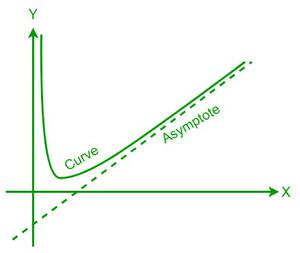### Asymptote Formula

Horizontal asymptote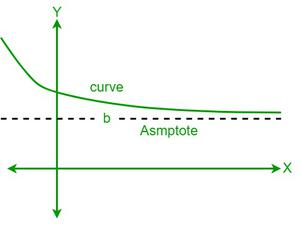The horizontal asymptotes are located where the curve approaches a constant value b as x approaches infinity (or negative infinity).

If f (x) = (axm +…)/(bxn +..) is a curve, its horizontal asymptotes are as follows:

1. If m < n, then the horizontal asymptote is y = 0, as x tends to infinity, i.e., limx⇢∞ f(x) = 0.
2. If m = n, then the horizontal asymptote is y = a/b, as x tents to infinity, i.e., limx⇢∞ f(x) = a/b.
3. If m > n, then the f(x) does not have a horizontal asymptote. limx⇢∞ f(x) = ±∞.

Vertical asymptote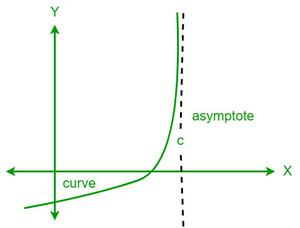A vertical asymptote is located when the curve shifts in the direction of infinity when x approaches a constant value c from the right or left.

So, to find the vertical asymptote of a function, its denominator must be equated to zero, as a function is undefined when its denominator is zero.

Oblique asymptote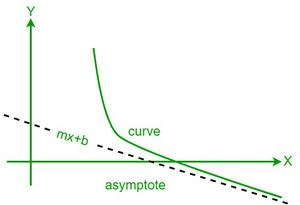An oblique asymptote occurs when the curve travels in the direction of the line y = mx + b while x also goes towards infinity in any direction.

Consider the function f(x) = p(x)/q(x), with p(x) and q(x) being polynomials. The given function will have an oblique asymptote only if the degree of the numerator is greater than the denominator. We get f(x) = a(x) + r(x)/q(x) by performing polynomial division on the given function, where a(x) is the quotient and r(x) is the reminder. Now, the oblique asymptote of the given function is a(x).

Asymptotes of a hyperbola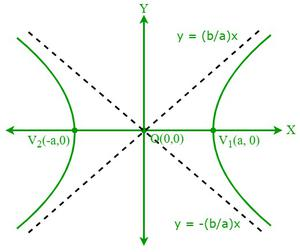A hyperbola has a pair of asymptotes having an equation x2/a2 – y2/b2 = 0.

Now, the equations of asymptotes are

Equation of asymptotes: y = (b/a) x and y = -(b/a) x

Equation of pair of asymptotes: x2/a2 – y2/b2 = 0.

### Sample Problems

Problem 1: If the equation of a hyperbola is x2/196 – y2/225 = 1, then find its asymptotes.

Solution:

Given,

The equation of the hyperbola is x2/196 – y2/225 = 1

If the equation of a hyperbola is x2/a2 – y2/b2 = 1, then the equation of its pair of asymptotes is x2/a2 – y2/b2 = 0

So, now the equation of the pair of asymptotes is x2/196 – y2/225 = 0

⇒ x2/(14)2 – y2/(15)2 = 0

⇒ (x/14 – y/15) (x/14 + y/15) = 0

⇒ (x/14 – y/15) = 0 and (x/14 + y/15) = 0

⇒ 15x – 14y = 0 and 15x + 14y = 0

Thus, the asymptotes of the given hyperbola are 15x – 14y = 0 and 15x + 14y = 0.

Problem 2: Find the vertical asymptotes for f(x) = 3x2 + 1/25x2 – 36.

Solution:

Given,

The equation of the curve f(x) = 3x2 + 1/25x2 – 36

We know that a vertical asymptote occurs when the curve tends to infinity.

So, the denominator should be equated to zero.

⇒ 25x2 – 36 = 0

⇒ (5x)2 – 62 = 0

⇒ (5x + 6) (5x – 6) = 0

⇒ x = -6/5 and x = 6/5

Hence, the vertical asymptotes are x = -6/5 and x = 6/5.

Problem 3: If the equation of a hyperbola is x2/64 – y2/4 = 0, then find its asymptotes.

Solution:

Given,

The equation of the hyperbola is x2/64 – y2/4 = 1

If the equation of a hyperbola is x2/a2 – y2/b2 = 1, then the equation of its pair of asymptotes is x2/a2 – y2/b2 = 0

So, now the equation of the pair of asymptotes is x2/64 – y2/4 = 0

⇒ x2/(8)2 – y2/(2)2 = 0

⇒ (x/8 – y/2) (x/8 + y/2) = 0

⇒ (x/8 – y/2) = 0 and (x/8 + y/2) = 0

⇒ 2x – 8y = 0 and 2x + 8y = 0

Thus, the asymptotes of the given hyperbola are 2x – 8y = 0 and 2x + 8y = 0.

Problem 4: Find the vertical asymptote of the curve f(x) = 5x2 – 4x + 1/x2 – 5x + 6.

Solution:

Given,

The equation of the curve f(x) = 5x2 – 4x + 1/x2 – 5x + 6

We know that a vertical asymptote occurs when the curve tends to infinity.

So, the denominator should be equated to zero.

⇒ x2 – 5x + 6 = 0

⇒ x2 – 2x – 3x + 6 = 0

⇒ (x – 2) (x – 3) = 0

⇒ x = 2 or x = 3

Hence, the vertical asymptotes are x = 2 and x = 3

Problem 5: Find the oblique asymptote of the curve f(x) = x2 + 8x – 15/x – 4

Solution:

Given,

The equation of the curve f(x) = x2 + 8x – 15/x – 4

The degree of the numerator is greater than denominator, so oblique asymptotes exits.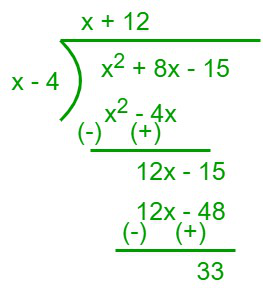Therefore, f(x) =  x2 + 8x – 15/x – 4 = (x + 12) + 33/(x – 4)

Hence, the oblique asymptote is y = x + 12.

Problem 6: What is the horizontal asymptote of the curve f(x) = x2 – 6x + 7/4x2 – 3?

Solution:

Given,

The equation of the curve f(x) = x2 – 6x + 7/4x2 – 3

Since, the degree of both numerator and denominator is equal, a horizontal asymptote exits.

To find, the horizontal asymptote, divide both numerator and denominator with x

f(x) = (x2 – 6x + 7/(x2)/(4x2 – 3)/(x2)

f(x) = (1 – 6/x + 7/x2)/(4 – 3/x2)

Now, limx⇢∞ f(x) = limx⇢∞ (1 – 6/x + 7/x2)/(4 – 3/x2)

= (1 – 0 + 0)/(4 – 0) = 1/4

Hence, the horizontal asymptote is y = 1/4

My Personal Notes arrow_drop_up